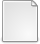# Computing the Action of the Matrix Exponential, with an Application to Exponential Integrators

Al-Mohy, Awad H. and Higham, Nicholas J. (2010) Computing the Action of the Matrix Exponential, with an Application to Exponential Integrators. [MIMS Preprint]There is a more recent version of this item available.PDF paper16.pdf Download (423kB)Other expmv.zip Download (8kB)

## Abstract

A new algorithm is developed for computing $e^{tA}B$, where $A$ is an $n\times n$ matrix and $B$ is $n\times n_0$ with $n_0 \ll n$. The algorithm works for any $A$, its computational cost is dominated by the formation of products of $A$ with $n\times n_0$ matrices, and the only input parameter is a backward error tolerance. The algorithm can return a single matrix $e^{tA}B$ or a sequence $e^{t_kA}B$ on an equally spaced grid of points $t_k$. It uses the scaling part of the scaling and squaring method together with a truncated Taylor series approximation to the exponential. It determines the amount of scaling and the Taylor degree using the recent analysis of Al-Mohy and Higham [\emph{SIAM J. Matrix Anal.\ Appl.} 31 (2009), pp.\ 970--989], which provides sharp truncation error bounds expressed in terms of the quantities $\|A^k\|^{1/k}$ for a few values of $k$, where the norms are estimated using a matrix norm estimator. Shifting and balancing are used as preprocessing steps to reduce the cost of the algorithm. Numerical experiments show that the algorithm performs in a numerically stable fashion across a wide range of problems, and analysis of rounding errors and of the conditioning of the problem provides theoretical support. Experimental comparisons with MATLAB codes based on Krylov subspace, Chebyshev polynomial, and Laguerre polynomial methods show the new algorithm to be sometimes much superior in terms of computational cost and accuracy. An important application of the algorithm is to exponential integrators for ordinary differential equations. It is shown that the sums of the form $\sum_{k=0}^p \varphi_k(A)u_k$ that arise in exponential integrators, where the $\varphi_k$ are related to the exponential function, can be expressed in terms of a single exponential of a matrix of dimension $n+p$ built by augmenting $A$ with additional rows and columns, and the algorithm of this paper can therefore be employed.

Item Type: MIMS Preprint To appear in SIAM Journal on Scientific Computing matrix exponential, Taylor series, ordinary differential equation, ODE, exponential integrator, $\varphi$ functions, backward error analysis, condition number, overscaling, Krylov method, Chebyshev polynomial, Laguerre polynomial, MATLAB, \texttt{expm}, CICADA MSC 2010, the AMS's Mathematics Subject Classification > 15 Linear and multilinear algebra; matrix theoryMSC 2010, the AMS's Mathematics Subject Classification > 65 Numerical analysis Nick Higham 06 Dec 2010 08 Nov 2017 18:18 http://eprints.maths.manchester.ac.uk/id/eprint/1536

### Actions (login required)View Item